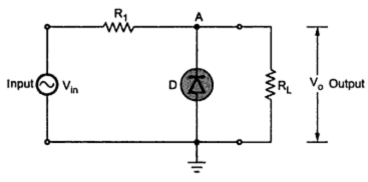### Basic Parallel Clipper with Negative Clipping

Parallel Clippers : Part3
Note : The negative clipping with basic parallel clipper can be achieved by reversing the direction of diode.
When Vin  is positive then the diode is reversed biased and acts as an open circuit. Thus the output voltage Vo is same as Vin  for R1 < < RL. Hence the positive half cycle of  Vin gets reproduced at the output. This is shown in the Fig. 1.Fig. 1  Basic parallel clipperFig. 2  Basic parallel clipper ( Negative clipping)
The magnitude of the output voltage is,
Consider the negative half cycle of Vin. As Vin decreases below zero and becomes -0.7 V, the diode becomes forward biased and acts conducting. As long as Vin < -0.7 V, the diode remains ON and drop across it is cut-in voltage Vγ = 0.7 V, with polarities as shown in the Fig. 2(a).
Note : As diode and RL are in parallel, the output voltage is equal to the drop across diode i.e. 0.7 V in opposite direction.
...                   Vo   = -0.7 V                           for Vin < -0.7V
Thus the entire negative half cycle gets clipped off, as shown in the Fig. 2(b).Fig.3  Basic parallel clipper ( Negative clipping)
The overall input-output waveforms can be shown as in the Fig. 3. The Fig.4(a) shows the waveforms with piecewise model of diode while the Fig. 4(b) shows the waveforms with an ideal diode approximation with Vγ = 0 V.Fig. 4
Transfer characteristics :
The transfer characteristics equations are,
The transfer characteristics are shown in the Fig. 5.Fig. 5  Transfer characteristics